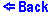Image Formation by a Converging Lens
You can move the object around by either clicking and draging or just clicking in the location of your choice. While the image stays real it appears on the right of the lens as a green arrow. When the image becomes virtual, it appeares on the left of the lens as a gray arrow. When the object is placed exactly at the focal point, the image appears at infinity.
The above applet shows: two arrows, a converging lens, and rays of light being emmitted by the red arrow. The red arrow is the object, while the green arrow is the image that results after the rays have passed through the lens. The applet also displays two focus shown as blue dots.

The image formed by a converging lens can be made using only three principal rays.

• Ray 1 is the ray which travels parallel to the axis and after going through the lens it passes through the focal point.
• Ray 2 passes through the center of the lens.
• Ray 3 goes through the focal point and then travels parallel to the axis after passing through the lens.
• Thus any point on the object can be mapped, using the rays above, into a corresponding point on the image. This point is located on the intersection of the rays.

The above is a useful tecnique, but it usually involves a drawing of some sort. A more practical way is not as complete but is much simpler. You can find the distance of the image from the lens by the following equation:

1/di + 1/do = 1/f ,
where di is the distance from the lens to the image, do is the distance from the object to the lens, and f is the focal distance of the lens.

Interesting things happen when do is equal to or greater than f. When you place the object directly at the focal point, solving the above equation for di we get:

di = 0,
i.e., no image results at all.

As the object is moved closer toward the lens, the image distance tends to zero from the negative side. This is called a virtual image represented by a gray arrow in this applet. Although the rays of light do not intersect, the mind perceives them to be coming from a point on the other side of the lens (this is shown by the dark green lines), which is infact the location of the virtual image.© Copyright 1997, Sergey Kiselev and Tanya Yanovsky-Kiselev